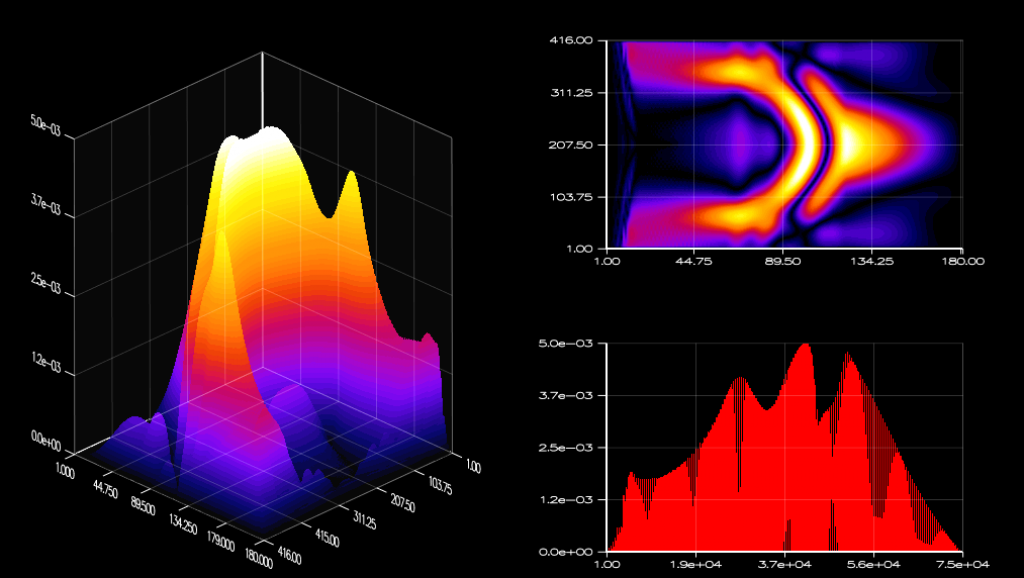# ArrayFire Examples (Part 7 of 8) - PDE

This is the seventh in a series of posts looking at our current ArrayFire examples. The code can be compiled and run from `arrayfire/examples/` when you download and install the ArrayFire library. Today we will discuss the examples found in the `pde/` directory.

In these examples, my machine has the following configuration:

```ArrayFire v1.9.1 (build XXXXXXX) by AccelerEyes (64-bit Linux)
CUDA toolkit 5.0, driver 319.17
GPU0 Tesla K20c, 5120 MB, Compute 3.5  (current)
GPU1 Tesla C2075, 6144 MB, Compute 2.0
GPU2 Tesla C1060, 4096 MB, Compute 1.3
Display Device: GPU0 Tesla K20c
Memory Usage: 5044 MB free (5120 MB total)```

The followings are the examples of formulating Partial Differential Equations, generally used to create a relevant computer model with several variables. In these examples, I don't introduce any new functions but focus on the performance of ArrayFire . You can experience the power of ArrayFire in these examples by looking at how it quickly handles the complex formulas and beautifully draws the result in real-time.

1. Shallow Water Equations - swe.cpp

This is a visualization of the shallow water flow below a pressure. The 2-D image in Figure 1 represents the wave propagation with boundary size of 512 x 512. The 3-D plot on the right in Figure 1 represents the gradient-versus-magnitude graph; it is not necessary to understand what each coordinate and the shape of the plot mean.

``` 21     unsigned io = (unsigned)floor(Lx / 5.0f),
22              jo = (unsigned)floor(Ly / 5.0f),
23              k = 20;
24
25     array x = tile(array(seq(nx),nx,1), 1,ny);
26     array y = tile(array(seq(ny),1,ny), nx,1);
27     array etam = 0.01f * exp((-((x - io) * (x - io) + (y - jo) * (y - jo))) / (k * k));
28     array eta = etam;
..
42     while (true) {
43
44         // compute
45         array up = um + convolve(eta, 2, diff_x_dims, h_diff_kernel);
46         array vp = um + convolve(eta, 2, diff_y_dims, h_diff_kernel);
47
48         array e = convolve(eta, 2, lap_dims, h_lap_kernel);
49         array etap = 2 * eta - etam + (2 * dt * dt) / (dx * dy) * e;
50         etam = eta;
51         eta = etap;```

Initially, a pressure is added to the shallow water as shown in line 21-28. Once the pressure is added, the flow of the shallow water is repeatedly splashed into many partial waves and destabilized as each wave reflects off the boundary. The Shallow Water Equation uses the zonal velocity, meridional velocity, acceleration due to gravity, force and etc., which makes the display look realistic. Along with these equations, all these physics math must be done fast enough to support the real-time updates in order to depict the waves quickly and smoothly for display.

```  iterations per second: 2984   (progress 5%)
iterations per second: 3348   (progress 10%)
...
iterations per second: 3340   (progress 90%)
iterations per second: 3339   (progress 95%)```

With our ArrayFire libraries, this example gets quick updates for every iteration, which occurs approximately 3300 iterations per second. ArrayFire also provides a expansion capability for drawing multiple objects in parallel manner.

2. Finite-Difference Time-Domain Simulation of Electromagnetic Field - fdtd.cppFinite-Difference Time-Domain is a numerical analysis technique to solve the electrodynamics problems. This is a cool visual demonstration of ArrayFire that contains various computations. It is not necessary to understand the details of this technique, but you may realize that the algorithm in the example is used to find a solution for very complicated problems, mostly handling differential equations.

```348         if (!console) {
349             // continually draw the current field
350             fig("color","heat");
351             fig("sub",2,2,2);   image(ey);
352             fig("sub",2,2,4);   plot2(ey);
353             fig("sub",2,1,1);   surface(ey);
354         }```

The image above includes three graphs in different dimensional perspectives. First, the SURFACE function - used for graph on the left - provides a three dimensional display with full 360 degree rotating camera view. The color layer changes by the height of the graph; the larger each value is the brighter its color appears to be, which provides a better vision for our determination. The perspective of IMAGE (top right) is visualized as if we are observing it from the top of the first graph. It's likely that the set of X and Y coordinates from the SURFACE graph without Z coordinate values; however, we can still relatively determine the height of each value by its different coloring. The graph on the bottom right is the side view of the first graph. As drawn, the graph displays with separating the overlapped graphics as much as possible by using filled and lined surface. Also, notice that the X-axis is different from other graphs; this is because PLOT2 takes the matrix data in a different way. So, there must be some adjustment needs for matrices to display one representation from different graphical views.

```Finite-difference time-domain simulation of electromagnetic field
iterations per second: 1643   (progress 7%)
iterations per second: 1635   (progress 10%)
iterations per second: 1627   (progress 13%)
iterations per second: 1625   (progress 17%)
...
iterations per second: 1619   (progress 90%)
iterations per second: 1616   (progress 93%)
iterations per second: 1614   (progress 97%)```

As you can see, the code is 400 lines and contains many computations. But the result still came out to be approximately 1620 iterations per second on my machine. This is a decent performance speed not only because it calculates the massive amount of values in matrices but also it runs them with smooth and fast graphics in real-time updates. If this algorithm had to be done in host (CPU), that could be a disaster for the performance in computation and display. Even if this could be done in device (GPU), without any library like ArrayFire, that could be another disaster for the efficiency in writing code. Thus, as users require writing a larger-scale program in GPU, ArrayFire is a more powerful tool to bring the best performance and efficiency.

If you consider yourself as a hardcore programmer, you may look for a powerful library. Download the ArrayFire library now and experience our useful functions with the best performance in your GPU.

---

Posts in this series: